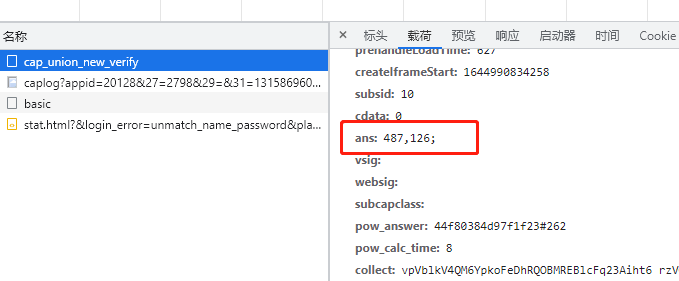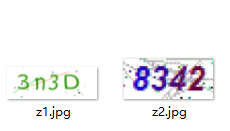#### 安装

 `1` ``````pip install ddddocr ``````

### 使用

#### 滑块验证码`12345678910111213` ``````import ddddocr det = ddddocr.DdddOcr(det=False, ocr=False) with open('hycdn.png', 'rb') as f: target_bytes = f.read() with open('background.jpg', 'rb') as f: background_bytes = f.read() res = det.slide_match(target_bytes, background_bytes, simple_target=True) print(res) ``````

 `1` ``````{'target_y': 0, 'target': [486, 126, 622, 262]} ``````

target 属性的前两个值正好和豆瓣验证滑块 url 中提交的 ans 差不多。#### 点选类验证码`1234567891011121314` ``````det = ddddocr.DdddOcr(det=True) with open("eb.jpg", 'rb') as f: image = f.read() poses = det.detection(image) im = cv2.imread("eb.jpg") for box in poses: x1, y1, x2, y2 = box im = cv2.rectangle(im, (x1, y1), (x2, y2), color=(0, 0, 255), thickness=2) cv2.imwrite("result.jpg", im) ``````#### 字母数字验证码`1234567` ``````ocr = ddddocr.DdddOcr(old=True) with open("z1.jpg", 'rb') as f: image = f.read() res = ocr.classification(image) print(res) ``````

 `12` ``````3n3d 8342 ``````

### 总结

ddddocr 让验证码变得如此简单与易用，,让不会用 opencv, pytorch, tensorflow 的小伙伴也能快速的破解网站的登录验证码。小伙伴们如果有其他好的 ocr 识别也可以在留言中分享出来。